PrecisionCalc
xl
Precision

Quick-Start Tutorial

## Installing xlPrecision

To install xlPrecision, simply download the file, double-click on it, and follow the instructions. Then, if Excel was running, exit and restart Excel.

Important:
On Windows NT, 2000, XP, Vista, 7 & 8, you must be logged in as an Administrator during installation. Start Setup by right-clicking xlPrecision.exe and choosing "Run as administrator":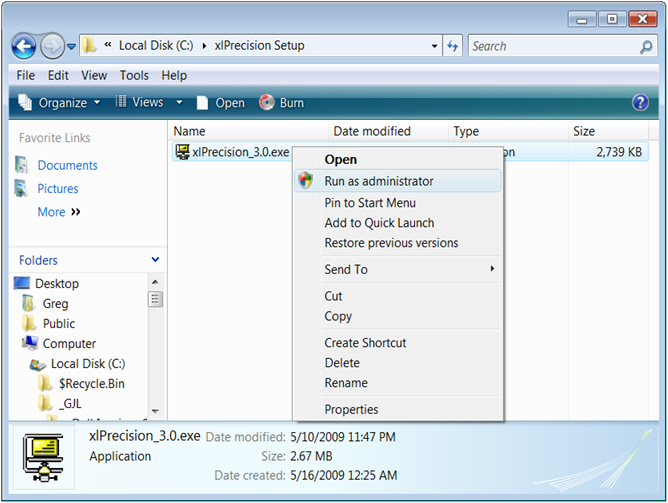After installation, you no longer need to be logged in as an Administrator.

After installing inspector text, start Excel (or restart Excel if it was already running).

To verify that xlPrecision is installed and working properly, enter this formula in any spreadsheet cell:

=xlpTEST()

It should return a welcome message with your name and the xlPrecision version. If it instead returns "#NAME!", then xlPrecision is not correctly installed. You may wish to try installing again, or see the Troubleshooting FAQ, or email for help.

Find xlPrecision's online help by choosing Add-Ins > xlPrecision 3.1 > xlPrecision 3.1 Help and Function Reference: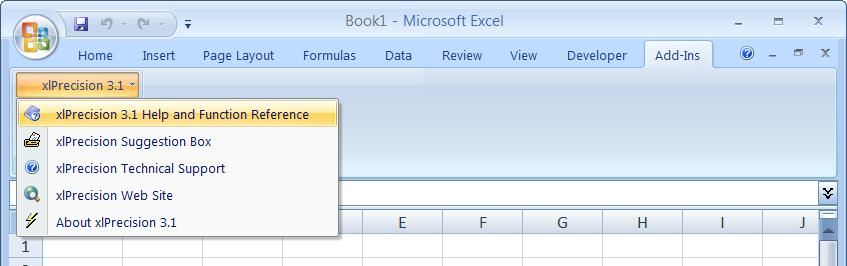In Excel 2003 and earlier, xlPrecision's online help is in Tools > xlPrecision 3.1 > xlPrecision 3.1 Help and Function Reference:## Using xlPrecision

xlPrecision provides high precision in Excel by replacing Excel's low precision arithmetic operators (+, - *, /, ^), comparison operators, (>, >=, <, <=, =), and some of its worksheet functions (SUM, MEDIAN, RANK, and many others), with custom worksheet functions, such as xlpADD, xlpMULTIPLY, xlpISLESS, xlpMEDIAN, etc. For a complete list of xlPrecision functions, see the Function Reference.

 Unfortunately, Excel does not allow changing the behavior of its built-in operators and worksheet functions, so it is not possible for xlPrecision to apply high precision to your existing worksheet formulas. Using xlPrecision requires modifying your worksheet formulas by replacing Excel's built-in operators with xlPrecision's custom worksheet functions. Of course, you only have to modify those formulas from which you want high precision.

For example, to get high precision from this worksheet formula:

=2/3

Change the formula to this:

=xlpDIVIDE(2,3)

 Be sure to put a comma (",") between the 2 and the 3, not a slash ("/").

 Depending on your world region and language, you might need to use a different character than the comma. Use whatever character you normally use to separate arguments in Excel functions.  In many locales, that character is a semi-colon (;).

The result should be as long as your edition of xlPrecision allows, or the default length of 100 significant digits, whichever is shorter. For example, if you are using the 25SD edition, the result should be 25 significant digits long, like this:

0.6666666666666666666666667

 xlPrecision results are returned as text that look like numbers, not as values that Excel recognizes as numbers. This is because Excel would immediately truncate the results to 15 significant digits if it recognized them as numbers.You can use the results of xlPrecision formulas as the operands in other xlPrecision formulas, but using them as operands in Excel's arithmetic functions will truncate them to 15 significant digits.

If you have a complex formula with more than one arithmetic operator or comparison operator, you may need to replace them all with xlPrecision functions. For example, in this formula, Excel discards the high precision returned by xlpDIVIDE when it evaluates the subtraction with Excel's built-in operator, the minus sign:

=xlpDIVIDE(A1,B1)-C1

To maintain high precision in that example, replace the minus sign with xlpSUBTRACT:

=xlpSUBTRACT(xlpDIVIDE(A1,B1),C1)

## Avoid Long Recalculations

When returning numbers with very high significant digits, or with very large operands, xlPrecision can take a long time to calculate. To prevent Excel from unnecessarily recalculating them, convert the affected formulas to text by copying them and then Paste Special > Values. And then be sure to save the workbook before closing it.

## Specifying Quantity of Significant Digits

If you don't specify how many significant digits you want, you'll get a maximum of 100, to prevent inadvertently starting long calculations. You can customize that maximum in the About box: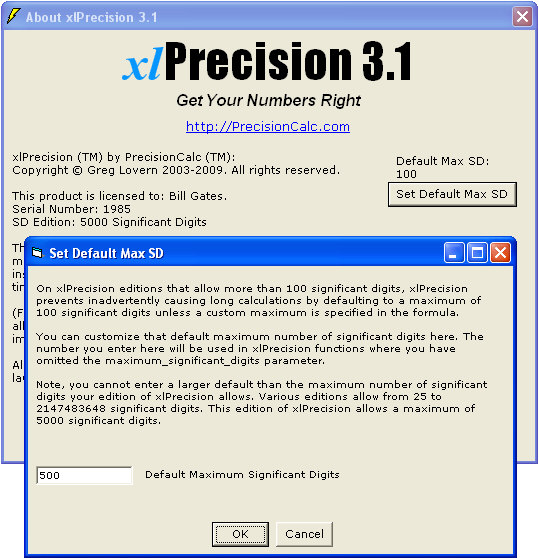For each xlPrecision function, you can specify any number of significant digits up to 32,767. For example, to get 1,000 significant digits, change the formula to this:

=xlpDIVIDE(2,3,,,,,1000)

If you specify more significant digits than your xlPrecision edition allows, then you'll get the maximum allowed by your edition.

Alternatively, you can specify fewer significant digits to speed up calculation.

## Other Arithmetic Operators

xlpADD(), xlpSUBTRACT(), xlpMULTIPLY(), xlpROOT(), and xlpPOWER() all work essentially the same way as xlpDIVIDE():

 Change this: To this: =2+3 =xlpADD(2,3) =2-3 =xlpSUBTRACT(2,3) =2*3 =xlpMULTIPLY(2,3) =2/3 =xlpDIVIDE(2,3) =2^(1/3) =xlpROOT(2,3) =2^3 =xlpPOWER(2,3)

The comparison operators are similar too:

 Change this: To this: =2=3 =xlpISEQUAL(2,3) =2<>3 =xlpISNOTEQUAL(2,3) =2>3 =xlpISGREATER(2,3) =2>=3 =xlpISGREATEROREQUAL(2,3) =2<3 =xlpISLESS(2,3) =2<=3 =xlpISLESSOREQUAL(2,3)

## Entering Operands

xlPrecision is capable of using operands (your input numbers) with up to 32,767 significant digits. But to prevent Excel from truncating them to 15 significant digits, you'll need to enter the operands as text instead of as a number:

• Right-click cell A1 and choose Format Cells,

• On the Number tab, in the Category listbox, select Text (not Number).

• In cell A1, enter 123456789123456789123456789.

• In cell A2, enter =xlpADD(A1,A1)

Alternatively, you can enter long operands within the formula by enclosing them with double-quotes so that Excel treats them as text rather than as numbers. For example, change the above formula to this:

## Formatting Options

Most xlPrecision functions also optionally allow:

• Formatting negative results with parentheses instead of a hyphen
• Formatting results with thousands separators (commas in the USA)
• Formatting with the local currency symbol
• Formatting the result in exponential notation
• Limiting the number of significant digits to make the calculation faster.
• Determining the number of decimal places.

For example, here's how it's done with xlpADD(), xlpSUBTRACT(), xlpMULTIPLY(), xlpDIVIDE(), xlpROOT(), and xlpPOWER():

 Argument Name Description Settings Default Example format_negative Determines whether negatives are formatted with a leading hyphen ("-"), or parentheses ("()"). Set to 1 to format negatives with a leading hyphen. Set to 2 for parentheses. You can also format negatives with a red font. 1 =xlpSUBTRACT(2,3,2)Returns "(1)" instead of default "-1". format_thousands Determines whether thousands separators are included. Set to TRUE to include thousands separators. FALSE =xlpMULTIPLY(200,300,,TRUE)Returns "60,000" instead of "60000" (or other thousands separator character depending on Region setting in Windows Control Panel). format_currency Determines whether currency symbol is included. Set to TRUE to include currency symbol. FALSE =xlpADD(2,3,,,TRUE)Returns "\$5" instead of "5" (or other currency symbol depending on Region setting in Windows Control Panel). exponential_notation Determines whether result is formatted in exponential notation. Set to TRUE to format result in exponential notation. FALSE =xlpADD(20000,300000,,,,TRUE)Returns "3.2E+5" instead of default "320000". maximum_significant_digits Determines the maximum number of significant digits to be returned. 1 - 32767 Depends on xlPrecision edition. =xlpDIVIDE(2,3,,,,,20)Returns 0.66666666666666666667. format_decimal_place Determines whether to add or remove decimal places as required to return the exact specified number of decimal places. 0 - 32767, or omitted Omitted =xlpDIVIDE(12.3,2,,,,,,4)Returns "6.1500" instead of default "6.15".

These optional arguments can be used in any combination desired. For example, this function:

=xlpDIVIDE(-20000,3,2,TRUE,TRUE,TRUE,20)

Returns this:

(\$6.66666666666666666666E+3)

Not all of these options are available with all xlPrecision editions. See the Buy page for a table showing which features are available with each edition.

You can also format negatives with a red font. See Format Negatives with Red Font.

 Many More Functions! See the xlPrecision Function Reference xlPrecision includes many other functions. Some xlPrecision functions use different syntax than shown above for their formatting options, and some have other options not shown here.  For detailed information on using each one, including exact examples showing how to use them, see the entries for each xlPrecision function.

## Insert Function Dialog

If you are using the 1,500 SD or higher edition, or the Free Edition, of xlPrecision, you can use Excel's Insert Function dialog to insert xlPrecision functions more conveniently than typing them in directly:

• Select the worksheet cell where you want the xlPrecision formula.
• Click the little "fx" to the left of Excel's formula bar. Or, click Formulas | Insert Function (in Excel 2003 and earlier, choose Insert | Function). The Insert Function dialog should appear.
• In the Insert Function dialog, bring down the Category dropdown, and select any of the High-Precision categories at the bottom of the list. The function list should show the xlPrecision functions in that category.
• Select a function and click OK. The Function Arguments dialog should appear for that function.
• For each of the argument fields, you can either type the argument in directly, or click the little square to the right of it, select a cell where you've put the desired argument. When you're done, click OK.
• If at any time you want to modify an xlPrecision formula, simply select that cell and click the little "fx" to the left of Excel's formula bar or choose Insert | Function. Instead of the Insert Function dialog, you'll then get the Function Arguments dialog, already filled out with the arguments you've already entered. Then, simply change them as desired and click OK.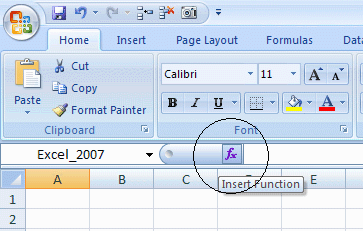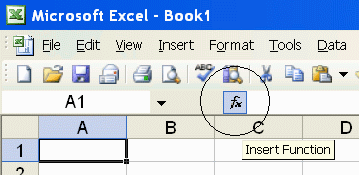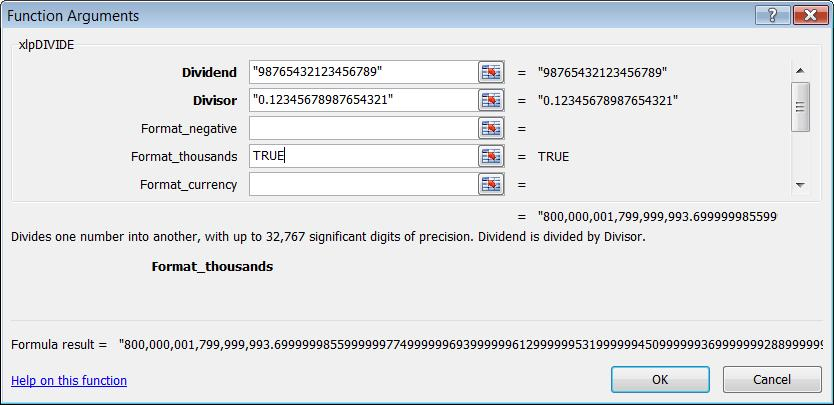Using the Insert Function dialog with xlPrecision functions requires the 1,500 SD or higher edition, or the Free Edition, of xlPrecision. Not recommended with the Free Edition as it causes the Free Edition dialog to appear multiple times.

 Using Excel's Insert Function Dialog with the xlPrecision Free Edition Using Excel's Insert Function dialog with the xlPrecision Free Edition is not recommended because it calculates on every keystroke, causing the Free Edition dialog to appear many times more than usual. Although it is not recommended in the Free Edition, it is enabled in the Free Edition so that you can evaluate it. If you do use it in the Free Edition, you might want to use Notepad to copy/paste whole arguments instead of typing them in, to minimize the number of times the Free Edition dialog appears.

Using Excel's Insert Function Wizard can be slow because Excel recalculates the function every time a digit or character is entered in the Wizard. To speed up that process, enter "0" for all numeric operands (such as num and rt for xlpROOT), so that the function does not take long to calculate. Then, when done with the Wizard, manually change the numeric operands to the desired numbers directly in the worksheet. Alternatively, you could use Notepad to copy/paste whole arguments instead of typing them in.

## Data Control & Analysis Features

xlPrecision offers many additional features that take you beyond Excel's capabilities in other ways. See Data Control & Analysis Features.

This has been a quick tutorial intended to get you started immediately. For more detail, please see the Main Tutorial:

Main Tutorial

For helpful tips on using xlPrecision, see:

Tips

For more detail on usage and syntax, please see:

Function Reference

For help on troubleshooting common problems, see:

Troubleshooting F.A.Q.

A mathematician is a person who says that when 3 people are supposed to be in a room but 5 come out, 2 have to go back in so the room can be empty.